Function Repository Resource:

# ApplyLevel

Create an operator that applies a function over data at a specified level

Contributed by: Seth J. Chandler
 ResourceFunction["ApplyLevel"][f,levelspec][data] applies the function f over data at the level set forth in levelspec.

## Details and Options

ResourceFunction["ApplyLevel"][f,levelspec] returns an expression with head Curry.
ResourceFunction["ApplyLevel"][f,{0}] is the same as Apply[f].

## Examples

### Basic Examples (2)

Create an Apply function that will apply a function f exclusively at parts of the expression lying at level 2:

 In:=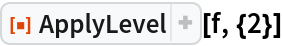Out=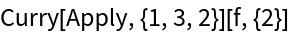Apply the function just created to an expression that is of depth 4:

 In:=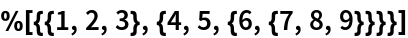Out=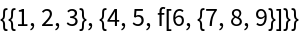### Scope (2)

ApplyLevel works with Association, just as Apply does:

 In:=In:=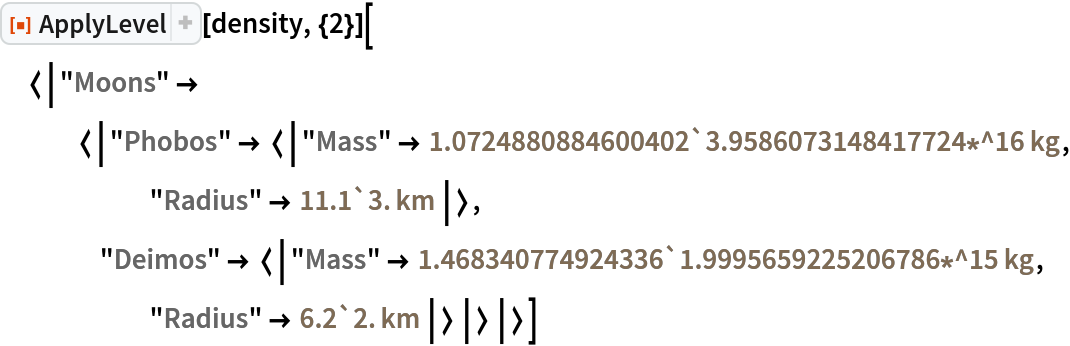Out=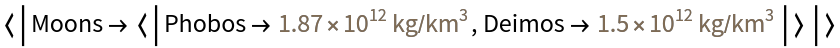ApplyLevel works on SparseArray objects just as it would on the corresponding ordinary lists:

 In:=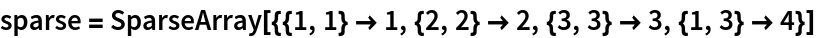Out=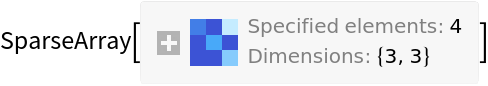In:=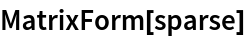Out=In:=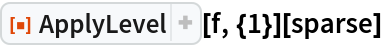Out=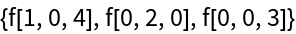### Applications (2)

Turn an Association in which the values are lists of vertex pairs into lists of undirected edges:

 In:=In:=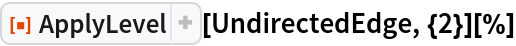Out=Now convert the result into a multigraph:

 In:=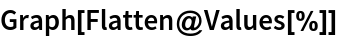Out=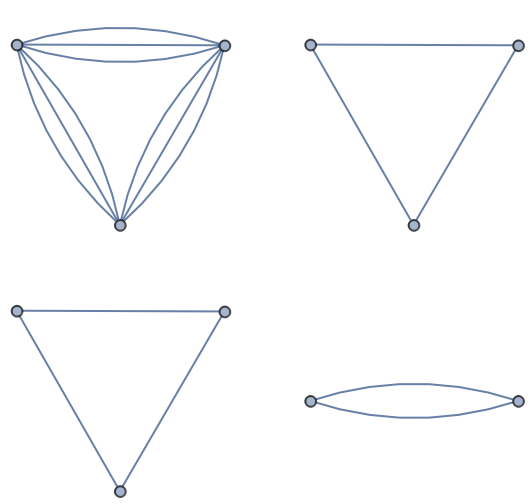### Neat Examples (2)

Compute the density of all the planetary moons, by first retrieving the planets Dataset:

 In:=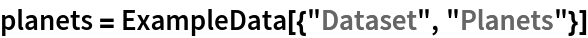Out=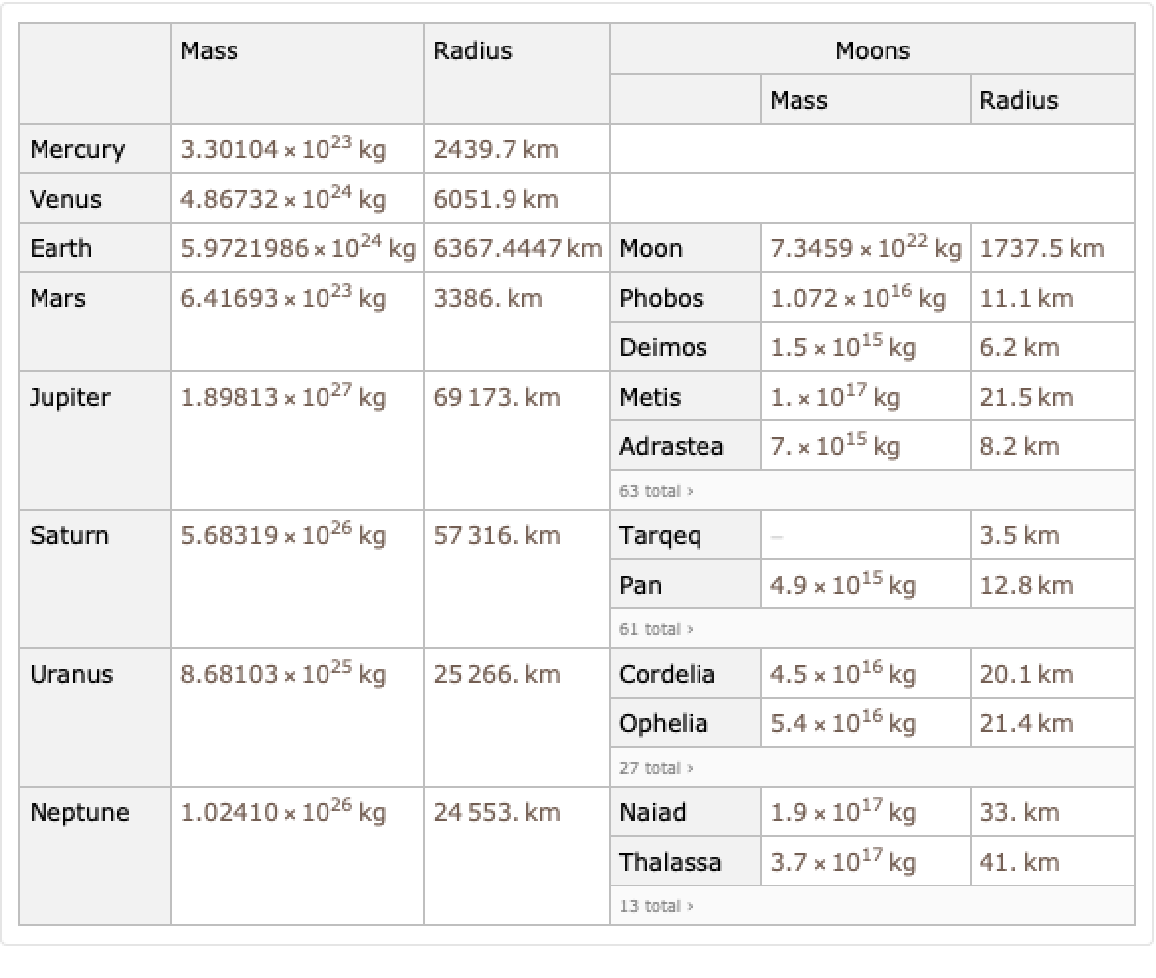Now use ApplyLevel as part of a pipeline of operators:

 In:=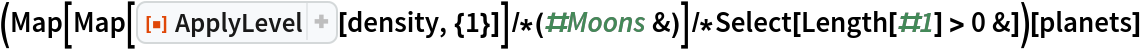Out=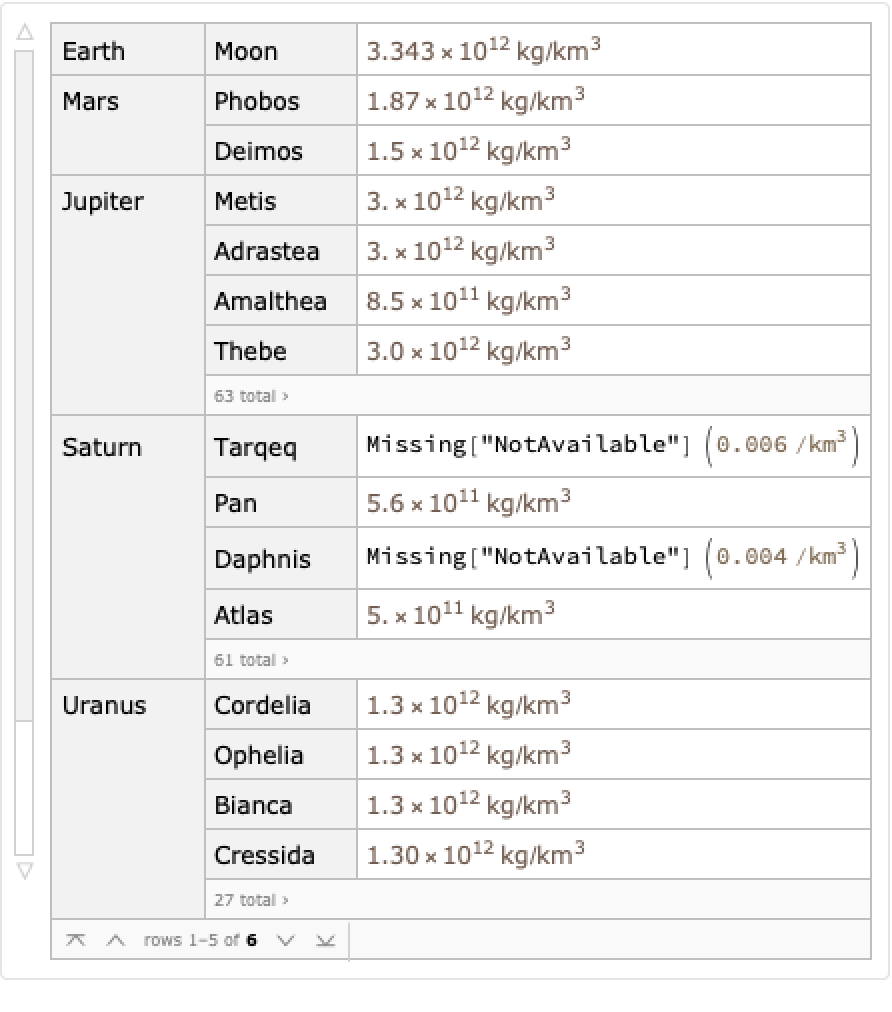Seth J. Chandler

## Version History

• 1.0.0 – 27 January 2020